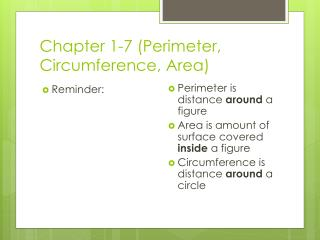Download PresentationChapter 1-7 (Perimeter, Circumference, Area)

# Chapter 1-7 (Perimeter, Circumference, Area) - PowerPoint PPT PresentationDownload Presentation## Chapter 1-7 (Perimeter, Circumference, Area)

- - - - - - - - - - - - - - - - - - - - - - - - - - - E N D - - - - - - - - - - - - - - - - - - - - - - - - - - -
##### Presentation Transcript

1. Chapter 1-7 (Perimeter, Circumference, Area) • Reminder: • Perimeter is distance around a figure • Area is amount of surface covered inside a figure • Circumference is distance around a circle

2. Chapter 1-7 (Perimeter, Circumference, Area) • Example • The in-bounds portions of a singles tennis court is shown. Find the perimeter and area.

3. Chapter 1-7 (Perimeter, Circumference, Area) • Example • The smallest circle on an Olympic target is 12 cm in diameter. Find the circumference and area.

4. Chapter 1-7 (Perimeter, Circumference, Area) • Example • Triangle JKL has vertices J(1, 6), K(6, 6), L(3, 2). Find the approximate perimeter of triangle JKL.

5. Chapter 1-7 (Perimeter, Circumference, Area) • Example • You are using a roller to smooth a lawn. You can roll about 125 yd2 in 1 minute. About how many minutes does it take to roll a lawn that is 120 ft. long and 75 ft. wide?

6. Chapter 1-7 (Perimeter, Circumference, Area) • Example • The base of a triangle is 24 ft. Its area is 216 ft2. Find the height of the triangle.